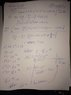# Using simpsons rule, how to find the error bound?

• Randall
In summary, the error bound for the Simpson's Rule approximation of the integral of sqrt(x) dx from 1 to 9 is 0.6667. To verify that the approximation is within this error bound, you can add the error bound to the calculated value and check if it falls within the actual value.

## Homework Statement

Find the error bound using Simpsons Rule for integral of SQRT (x) dx from 1 to 9.[/B]

## Homework Equations

E = (M * (b-a)^5) / (180 * n^4), where M = max value of the 4th deriv of x dx
[/B]

## The Attempt at a Solution

see attached please - I can't figure out what to use for the value of M, which is supposed to be the maximum value of the 4th derivative. I graphed the 4th deriv (see attached) and the max y = 0 and the max x is infinity? I need help figuring out M please thanks.https://www.dropbox.com/s/3cn5q3n96iutgu4/IMG_6352.JPG?dl=0 [/B]

#### Attachments

•IMG_6352.JPG
28.7 KB · Views: 766
Randall said:

## Homework Statement

Find the error bound using Simpsons Rule for integral of SQRT (x) dx from 1 to 9.[/B]

## Homework Equations

E = (M * (b-a)^5) / (180 * n^4), where M = max value of the 4th deriv of x dx
[/B]

## The Attempt at a Solution

see attached please - I can't figure out what to use for the value of M, which is supposed to be the maximum value of the 4th derivative. I graphed the 4th deriv (see attached) and the max y = 0 and the max x is infinity? I need help figuring out M please thanks.https://www.dropbox.com/s/3cn5q3n96iutgu4/IMG_6352.JPG?dl=0 [/B]

M is not the max value of the fourth derivative of x dx (although it is related to the fourth derivative of ##f(x)## = the integrand in ##\int_a^b f(x) \, dx##). In fact,
$$M = \max_{a \leq x \leq b} |f^{(4)} (x) |$$

What happens near x = 0 or out at x = ∞ is irrelevant, since the only values the matter here are the ones between x = 1 and x = 9.

Assuming the 1/3 rule, the approximate error is given by:

$$E_a = - \frac{(b-a)^5}{180n^4} \space \bar f^{(4)}(\epsilon)$$

Where ##\bar f^{(4)}(\epsilon) = \frac{\int_a^b f^{(4)}(x) \space dx}{b - a}## is the average fourth derivative on the interval.

Ray Vickson said:
M is not the max value of the fourth derivative of x dx (although it is related to the fourth derivative of ##f(x)## = the integrand in ##\int_a^b f(x) \, dx##). In fact,
$$M = \max_{a \leq x \leq b} |f^{(4)} (x) |$$

What happens near x = 0 or out at x = ∞ is irrelevant, since the only values the matter here are the ones between x = 1 and x = 9.

Thank you!
-R

Zondrina said:
Assuming the 1/3 rule, the approximate error is given by:

$$E_a = - \frac{(b-a)^5}{180n^4} \space \bar f^{(4)}(\epsilon)$$

Where ##\bar f^{(4)}(\epsilon) = \frac{\int_a^b f^{(4)}(x) \space dx}{b - a}## is the average fourth derivative on the interval.

Thank you!
-R

Ok. So after calculating the error bound using M=15/16, I get 0.6667. Does this mean the approximation needs to be within 0.66% of actual? Or does it need to be within 66.67% of actual? 66.67% seems awfully big, and 0.66% seems to be awfully small...

Last edited:
Randall said:
Ok. So after calculating the error bound using M=15/16, I get 0.6667. Does this mean the approximation needs to be within 0.66% of actual? Or does it need to be within 66.67% of actual?
Neither. What makes you think it's a fractional or percentage error?

Oh! Ok. So what do I do with this 0.6667 value then? What does that tell me? The problem wants to verify that the approximate value (found via the Simpsons rule) of 17.32 is within the error bound (0.6667) of the actual value of 17.6667. Do I add 0.6667 to 17.32 = 18, and since the actual value is 17.6667 (which is less than 18), I am within the error bound?

Randall said:
Oh! Ok. So what do I do with this 0.6667 value then? What does that tell me? The problem wants to verify that the approximate value (found via the Simpsons rule) of 17.32 is within the error bound (0.6667) of the actual value of 17.6667. Do I add 0.6667 to 17.32 = 18, and since the actual value is 17.6667 (which is less than 18), I am within the error bound?
Yes. Logically you would run that the other way: is the calculated value between (actual - bound) and (actual + bound), but it comes to the same thing.